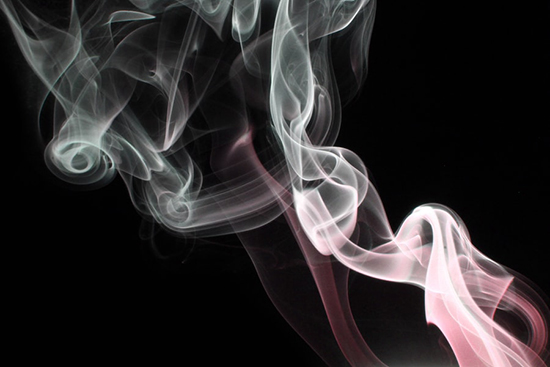Gases are made of billions of energetic molecules that collide with and interact with each other. Since they behave differently at different temperatures and pressures, it is often difficult to assign exact empirical values to them. To overcome this problem, it was necessary to imagine a gas that is amenable to statistical analysis. Thus was born the concept of ideal gases.
Definition of an ideal gas
An ideal gas is a theoretical (conceptual) gas that abides by the gas laws (there are several of them, such as Boyle’s Law) and has the following properties:

• The molecules of this gas neither attract nor repel each other. The only way they interact is by an elastic collision with each other or with the walls of the container.
• The molecules of this gas do not have volume. In other words, they do not occupy any space. The gas itself has volume, but not its molecules.

A tall order, you might say. But ideal gases are not completely fictitious. A majority of gases – including nitrogen, hydrogen and oxygen – behave nearly like an ideal gas at the correct temperature and pressure.
Ideal Gas Law
The relationship between the pressure, volume and temperature of an ideal gas can be expressed by a simple mathematical equation. This is known as the ideal gas law or general gas equation.
PV = nRT
Where P is the pressure, V is the volume, T is the temperature, n is the amount of the substance in the gas in moles, and R is the ideal gas constant.
The gas constant, denoted by R, is a physical constant whose value is 8.3144598(48) J?mol?1?K?1. The value of R can also be expressed as 0.082 L.atm / K.mol.
1 mole of ideal gas has a volume of 22.710947 litres at standard temperature and pressure.
If you use the unit 8.3144598(48) J?mol?1?K?1 for R, then you must use the value of P in Pascal, V in m3, and T in kelvin. But if you use 0.082 L.atm/K.mol, you must use the value of P in atm, V in litres, and T in kelvin.
That was the molar form of the ideal gas law. In molecular form, the law is expressed as:
PV = NkBT
Where N is the number of molecules in the gas and kB is Boltzmann’s constant whose value is 1.38 x 10-23 JK-1. Here, the pressure P must be in Pascal, volume V in m3, and temperature T in kelvin.
The ideal gas law gives a good approximation of how a gas behaves under different conditions. It is actually a combination of Boyle’s Law, Charles’ Law and Avogadro’s Law.

• Boyle’s law states that at any given temperature and for any given number of moles, the volume of a given mass of gas is inversely proportional to the pressure exerted on it.
• Charles’ law states that assuming a closed system, the volume of a given mass of gas at constant pressure is directly proportional to its absolute temperature.
• Avogadro’s law states that the volume of an ideal gas present in a container is directly proportional to the number of molecules of the gas present in it.

As you can see, the ideal gas law expressed by molar equation PV = nRT encompasses all these three laws. If you found this hard to understand, then you may need tuition in physics. When choosing a physics tuition centre, make sure that they have qualified and experienced tutors.